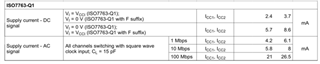If you have a related question, please click the "Ask a related question" button in the top right corner. The newly created question will be automatically linked to this question.

# ISO7763-Q1: How to estimate the power consumption of by the supply current

Part Number: ISO7763-Q1
Other Parts Discussed in Thread: ISO7763

Hi Expert,

My customer is using the ISO7763-Q1 in the Traction Inverter platform. And they have the question on the calculation of power consumption.

1. The data sheet has the below informationFor the DC and AC supply current, is that refer to the one channel operation? Can I said that, if the 6 channel are used, the total supply current is the Isupply*6?

2. In the datasheet, it also has the total power dissipation as the reference, is that calculate in the case of all channel are used?

Thank you!

Pin

• Hi Pin,

Thanks for reaching out, please see my inputs below.

For the DC and AC supply current, is that refer to the one channel operation? Can I said that, if the 6 channel are used, the total supply current is the Isupply*6?

ICC1 listed in the datasheet is the total current consumed by VCC1 and hence, is the primary side current of all 6-channels. Similarly, ICC2 is the total current going into VCC2. ICC1 + ICC2 will give you total current consumption of the whole device including primary and secondary by all 6-channels.

2. In the datasheet, it also has the total power dissipation as the reference, is that calculate in the case of all channel are used?

I assuming you are referring to section 6.5 in ISO7763 datasheet titled "Power Ratings". This is power rating, which means that this is the maximum power dissipation allowed in the device. This is the maximum that the device dissipates under wort-case operating conditions. Thanks.

Regards,
Koteshwar Rao